9th Class Science Notes in English chapter 8 Motion

CBSE Revision Notes for CBSE Class 09 Science Motion Motion: Distance and displacement, velocity; uniform and non-uniform motion along a straight line; acceleration, distance-time and velocity-time graphs for uniform motion and uniformly accelerated motion, derivation of equations of motion by graphical method; elementary idea of uniform circular motion.

Class 9th Science chapter 8 Motion Notes in English.

📚 Chapter - 8 📚
👉 Motion 👈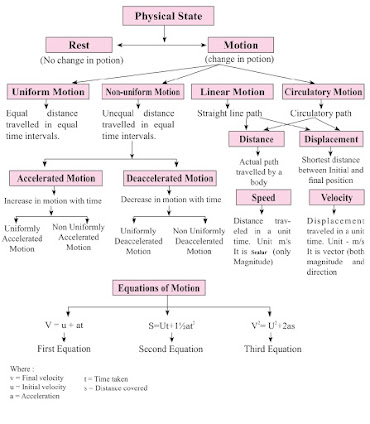✳️Rest:-

🔹 A body is said to be in a state of rest when its position does not change with respect to a reference point

✳️Motion:-

🔹 A body is said to be in a state of motion when its position change continuously with reference to a point.

✳️Motion Along a Straight Line:-

🔹 When an object moves along a straight line, the motion of the object is called rectilinear motion. For example; motion of a car on highway.

✳️Scalar Quantity:-

🔹 It is the physical quantity having own magnitude but no direction
e.g., distance, speed.

✳️Vector Quantity:-

🔹 It is the physical quantity that requires both magnitude and direction
e.g., displacement, velocity.

✳️Distance:-

🔹 The actual path or length travelled by a object during its journey from its initial position to its final position is called the distance.
Distance is a scalar quantity which requires only magnitude but no direction to explain it.

✳️Displacement:-

🔹 It is the minimum distance from initial position to final position.
Displacement is a vector quantity requiring both magnitude and direction for its explanation. It can be zero (when initial point and final point of motion are same)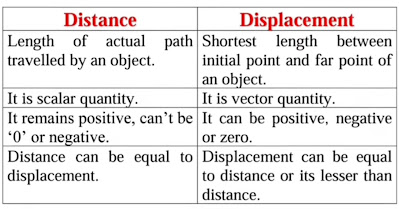There are Two types of Motion:-

✳️Uniform motion:-

🔹 When an object covers equal distances in equal intervals of time, it is said to be in uniform motion.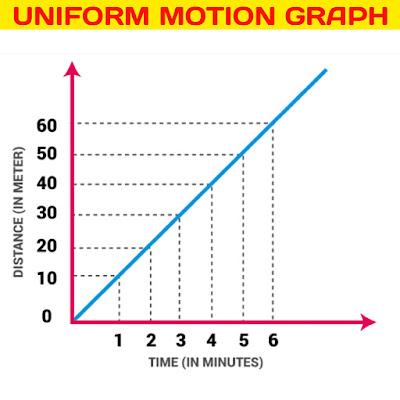✳️Non-uniform motion:-

🔹 Motions where objects cover unequal distances in equal intervals of time.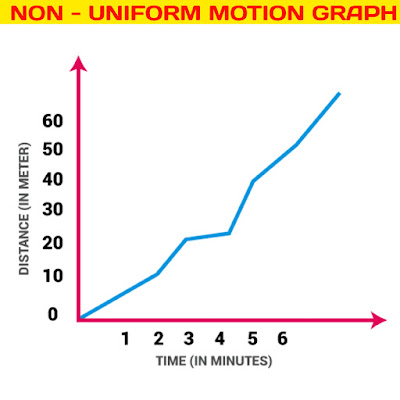🔹 Non-uniform motion is of two types:-

👉 (i) Accelerated Motion:- When motion of a body increases with time.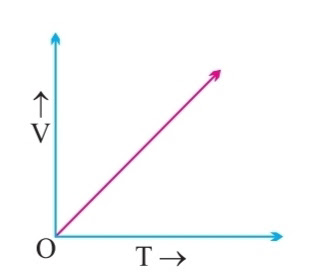👉 (ii) De-accelerated Motion:- When motion of a body decreases with time,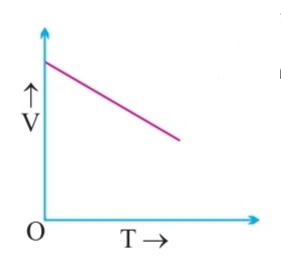✳️Speed:-

🔹 The measurement of distance travelled by a body per unit time is called speed.
👉Speed= Distance travelled / Time taken
👉SI Unit= m/s (meter/second)

🔹 If a body is executing uniform motion, then there will be a constant speed or uniform motion.

🔹 If a body is travelling with non-uniform motion, then the speed will not remain uniform but have different values throughout the motion of such body.

🔹 For non-uniform motion, average speed will describe one single value of speed throughout the motion of the body.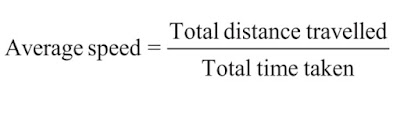👉Average Speed= Total Distance / Total time

✳️Velocity:-

🔹 It is the speed of a body in given direction.

👉Velocity= Displacement / Time
👉SI Unit of velocity = ms'⁻¹

🔹 Velocity is a vector quantity. Its value changes when either its magnitude or direction changes.

🔹 For non-uniform motion in a given line, average velocity will be calculated in the same way as done in average speed.

👉Average velocity= Total Displacement / Total time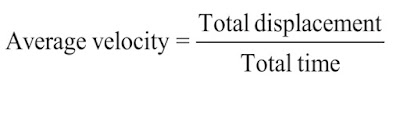🔹 For uniformly changing velocity, the average velocity can be calculated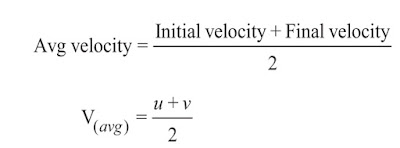👉Average Velocity= u+v /2
u= Initial Velocity
v= Final Velocity

✳️Acceleration:-

🔹 Acceleration is seen in non-uniform motion and it can be defined as the rate of change of velocity with time.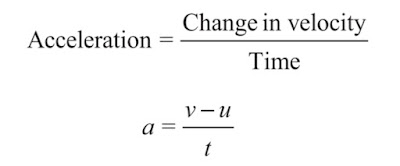👉Acceleration= Change in velocity/Change in time
a = v-u /t

👉SI Unit= ms⁻²

✳️Retardation/Deacceleration:-

🔹 Deaceleration is seen in non-uniform motion during decrease in velocity with time. It has same definition as acceleration.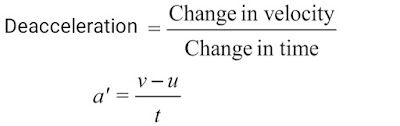👉Deaceleration= Change in velocity/Change in Time

a'= (v-u)/t
Here, v < u, ‘a’ = negative (-ve).

✳️Graphical representation of motions:-

👉(i) Distance-time graph:-

🔹 For a distance-time graph, time is taken on x-axis and distance is taken on the y-axis.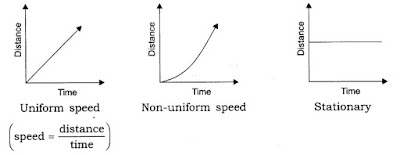👉(ii) Velocity-time graph:-

🔹 For a Velocity-time graph, time is taken on x-axis and Velocity is taken on the y-axis.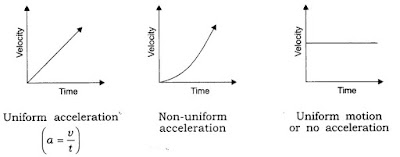✳️Equation of motion by graphical methods:-

👉(i) velocity-time relation (v = u + at):-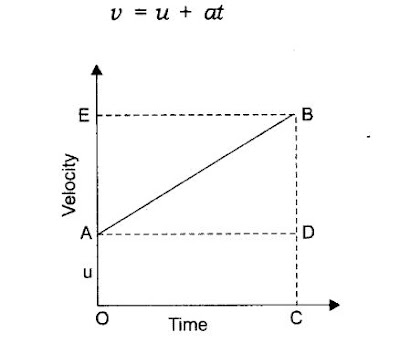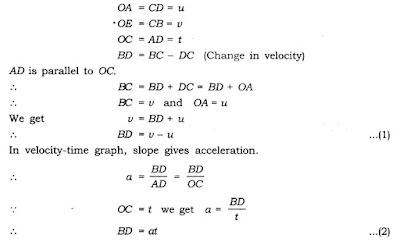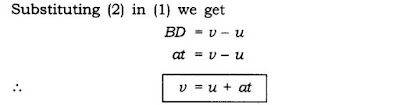👉(ii) The equation for position-time relation (S = ut + ½at²):-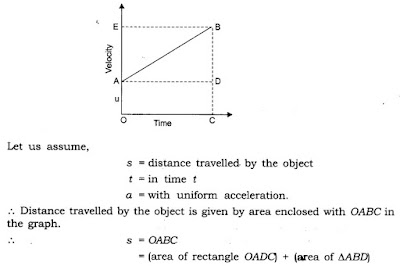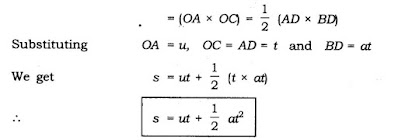👉(iii) Equation for position-velocity relation (v² = u² + 2as):-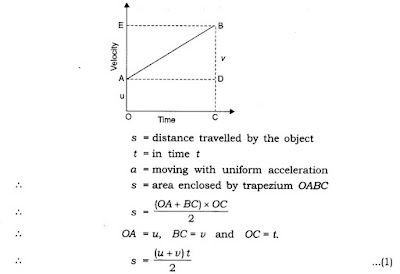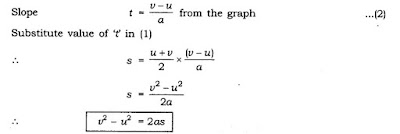✳️Uniform Circular Motion:-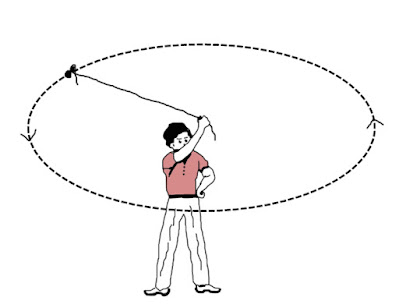🔹 If a body is moving in a circular path with uniform speed, then it is said to be executing uniform circular motion.

🔹 In such a motion the speed may be same throughout the motion but its velocity is different at each and every point of its motion. Thus, uniform circular motion is an accelerated motion.